Next: Precession Up: Useful mathematics Previous: Conservative fields

# Rotational coordinate transformations

Consider a conventional right-handed Cartesian coordinate system,,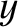,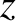. Suppose that we transform to a new coordinate system,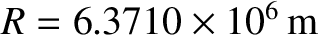,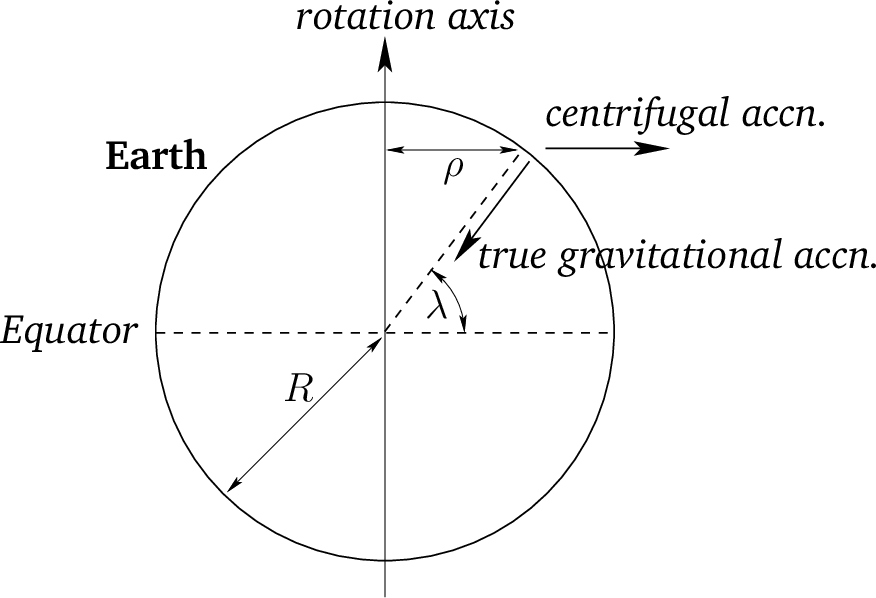,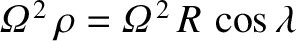, that is obtained from the,,system by rotating the coordinate axes through an angle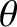about the-axis. (See Figure A.1.) Let the coordinates of a general point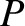be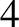in the first coordinate system, and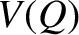in the second. According to simple trigonometry, these two sets of coordinates are related to one another via the transformation: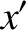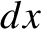(A.86)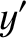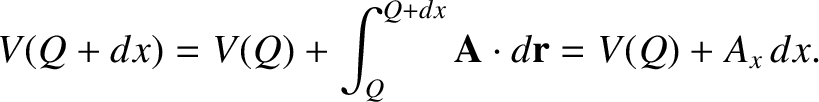(A.87) and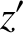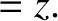(A.88)

When expressed in matrix form, this transformation becomes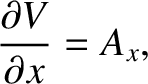(A.89)

The reverse transformation is accomplished by rotating the coordinate axes through an angle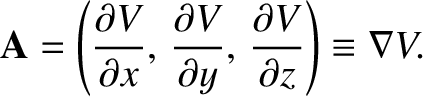about the-axis: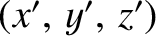(A.90)

It follows that the matrix appearing in Equation (A.89) is the inverse of that appearing in Equation (A.90), and vice versa. However, because these two matrices are clearly also the transposes of one another, we deduce that both matrices are unitary. In fact, it is easily demonstrated that all rotation matrices must be unitary; otherwise they would not preserve the lengths of the vectors on which they act.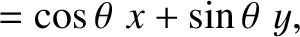A rotation through an angle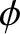about the-axis transforms the,,coordinate system into the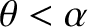,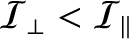,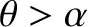system, where, by analogy with the previous analysis,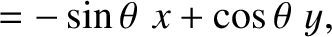(A.91)

Thus, from Equations (A.89) and (A.91), a rotation through an angleabout the-axis, followed by a rotation through an angleabout the-axis, transforms the,,coordinate system into the,,system, where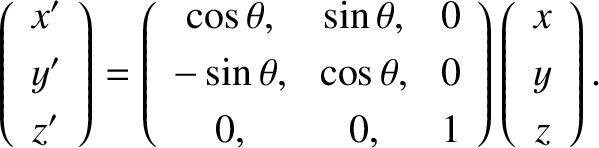(A.92)Next: Precession Up: Useful mathematics Previous: Conservative fields
Richard Fitzpatrick 2016-03-31